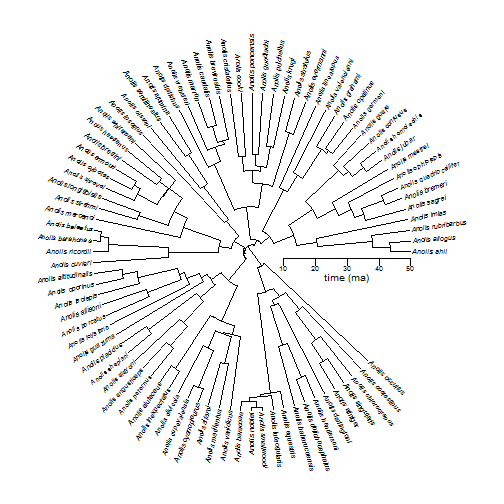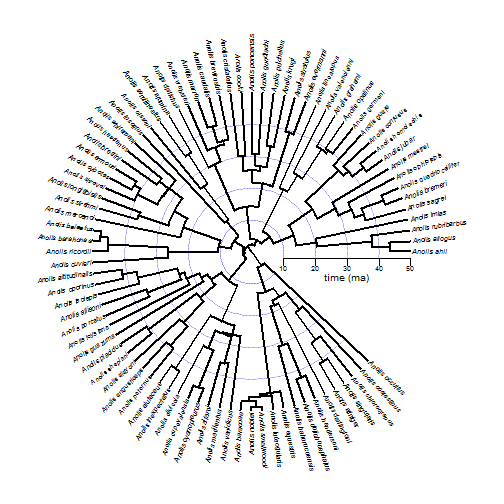## Tuesday, September 26, 2017

### Plotting a fan-style (circular) tree with a time axis

I don't know if I've ever shown this before, but here is an example of a fan style (circular) tree with a time axis:

``````library(phytools)
data(anoletree)
## rescale it to 50 units
tree<-phytools:::rescaleTree(anoletree,50)
plotTree(tree,ftype="i",lwd=1,fsize=0.6,type="fan",part=0.88)
obj<-axis(1,pos=-2,at=seq(10,50,by=10),cex.axis=0.5,labels=FALSE)
text(obj,rep(-5,length(obj)),obj,cex=0.6)
text(mean(obj),-8,"time (ma)",cex=0.8)
``````Here's another feature that could be neat to add using `plotrix::draw.arc`:

``````plotTree(tree,ftype="i",lwd=1,fsize=0.6,type="fan",part=0.88)
obj<-axis(1,pos=-2,at=seq(10,50,by=10),cex.axis=0.5,labels=FALSE)
text(obj,rep(-5,length(obj)),obj,cex=0.6)
text(mean(obj),-8,"time (ma)",cex=0.8)
for(i in 1:(length(obj)-1)){
a1<-atan(-2/obj[i])
a2<-0.88*2*pi
col=make.transparent("blue",0.25))
}
plotTree(tree,type="fan",
xlim=get("last_plot.phylo",envir=.PlotPhyloEnv)\$x.lim,
ylim=get("last_plot.phylo",envir=.PlotPhyloEnv)\$y.lim,